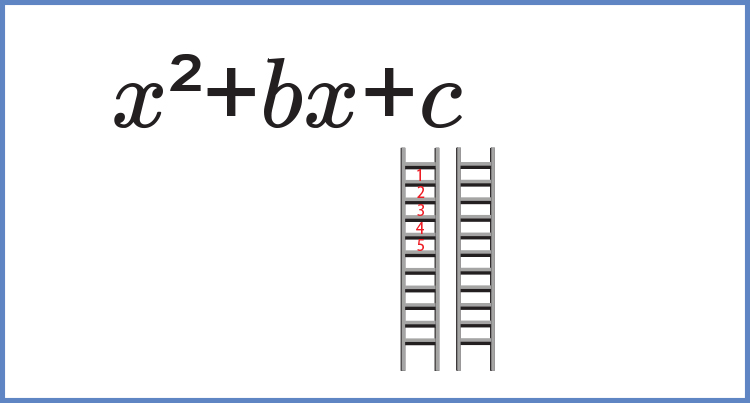## (The third of three ways to solve (find the roots) of quadratics)

We know factors are found using ladders, and we know quadratics have an x^2  term.

To factor a general quadratic formula as

x^2+bx+c=0Find factors of c  that add to give b.